Dot Products and Projections

The Dot Product (Inner Product)

There is a natural way of adding vectors and multiplying vectors by scalars. Is there also a way to multiply two vectors and get a useful result? It turns out there are two; one type produces a scalar (the dot product) while the other produces a vector (the cross product). We will discuss the dot product here.

The dot product of two vectors a=<a_1,a_2,a_3> and b=<b_1,b_2,b_3> is given by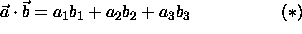An equivalent definition of the dot product is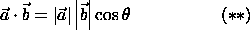where theta is the angle between the two vectors (see the figure below) and |c| denotes the magnitude of the vector c. This second definition is useful for finding the angle theta between the two vectors.#### Example

The dot product of a=<1,3,-2> and b=<-2,4,-1> is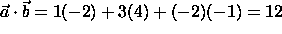Using the (**)we see thatwhich implies theta=45.6 degrees.

An important use of the dot product is to test whether or not two vectors are orthogonal. Two vectors are orthogonal if the angle between them is 90 degrees. Thus, using (**) we see that the dot product of two orthogonal vectors is zero. Conversely, the only way the dot product can be zero is if the angle between the two vectors is 90 degrees (or trivially if one or both of the vectors is the zero vector). Thus, two non-zero vectors have dot product zero if and only if they are orthogonal.

#### Example

<1,-1,3> and <3,3,0> are orthogonal since the dot product is 1(3)+(-1)(3)+3(0)=0.

One important use of dot products is in projections. The scalar projection of b onto a is the length of the segment AB shown in the figure below. The vector projection of b onto a is the vector with this length that begins at the point A points in the same direction (or opposite direction if the scalar projection is negative) as a.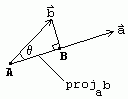Thus, mathematically, the scalar projection of b onto a is |b|cos(theta) (where theta is the angle between a and b) which from (*) is given by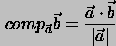This quantity is also called the component of b in the a direction (hence the notation comp). And, the vector projection is merely the unit vector a/|a| times the scalar projection of b onto a: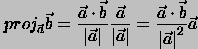Thus, the scalar projection of b onto a is the magnitude of the vector projection of b onto a.

#### Example

Suppose you wish to find the work W done in moving a particle from one point to another. From physics we know W=Fd where F is the magnitude of the force moving the particle and d is the distance between the two points. However, this relation is only valid when the force acts in the direction the particle moves. Suppose this is not the case. Let the force vector be F=<2,3,4> and the displacement vector be d=<1,2,3>. In this case, the work is the product of the distance moved (the magnitude of the displacement vector) and the magnitude of the component of the force that acts in the direction of displacement (the scalar projection of F onto d):Thus, the work done by the force to displace the particle from say the origin to the point (1,2,3) is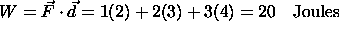Note that this is the easiest way to compute the dot product since the angle between the vectors F and d is unknown.